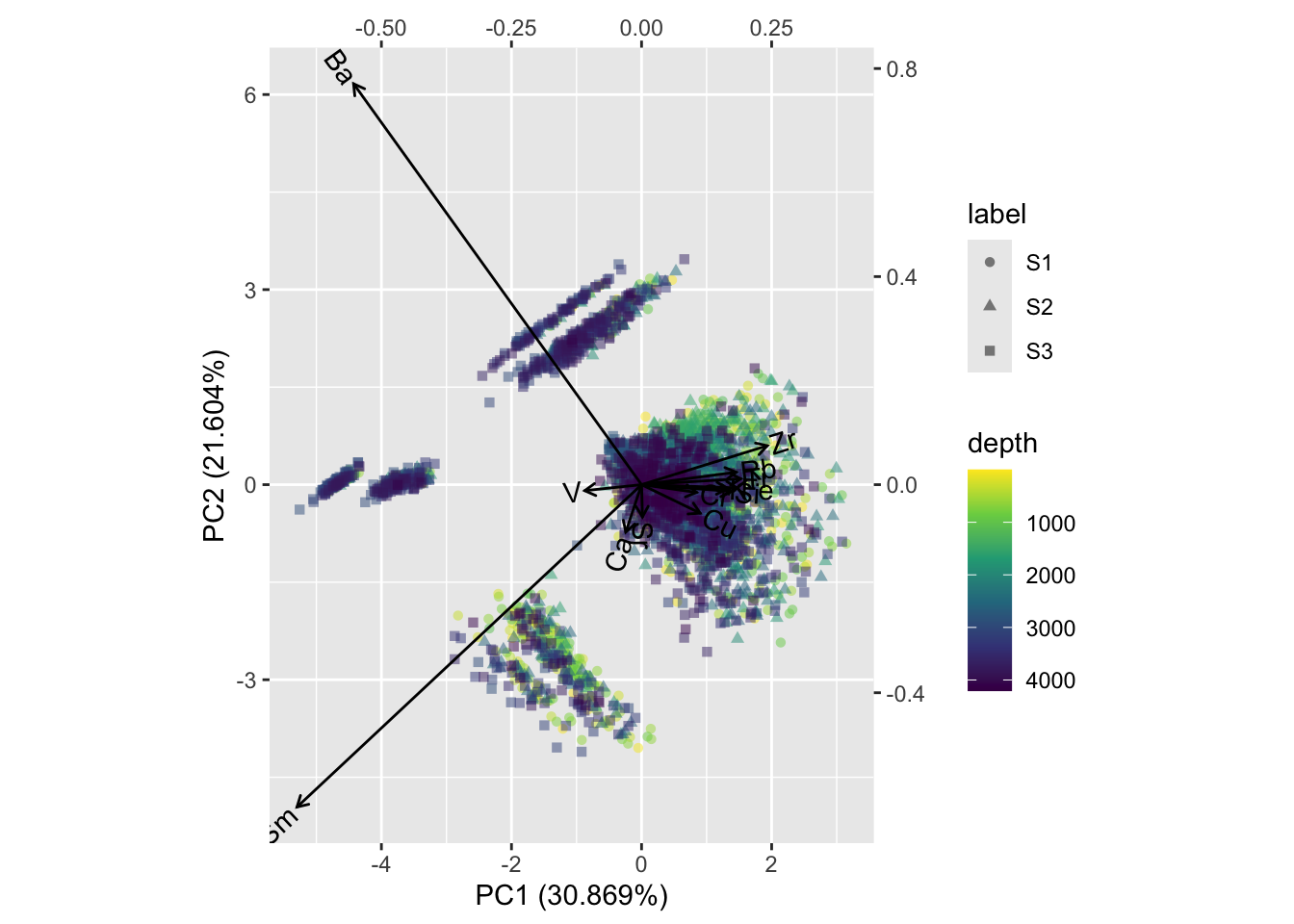## 6.3 Using `ordr` for PCA

The `ordr` package can be used to retain metadata (depths, labels, groups etc.) along with the PCA object. This is particularly useful for complex biplots using `ggplot2`. The following example uses the `CD166_19_xrf_acomp_meta` from the previous section; note that `myElements` is a vector of elements to include in the ordination, in this case generated in the section Noisy Data.

``````CD166_19_xrf_acomp_meta %>%
ordr::ordinate(., cols = any_of(myElements),
model = ~ princomp(clr(.)),
augment = any_of(c("depth", "label"))
) %>%

ordr::ggbiplot(., sec.axes = "cols", scale.factor = 8) +
ordr::geom_rows_point(aes(colour = depth, shape = label), alpha = 0.5) +
ordr::geom_cols_vector() +